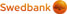Navigate to main page content

# Option Calculator

In its present shape the calculator is a research and learning tool, which makes it possible for an investor to calculate the approximate option premium. As a bonus, the calculator displays some of the more noteworthy ratios, which influence the premium. The actual parameters of an option contract are agreed by the contracting parties.

Freely chosen numbers, including decimals, may be entered into the calculator's fields. Even a premium for a Thai baht-nominated contract on Indian rupee can be calculated, though there might be a long journey to the nearest counterparty for the contract.

Current Price
Strike
Interest Rate (% p.a.)
dividend
Volatility (% p.a.)
Units Left/End Date

Manual

You can enter the following factors into the calculator:

Current price is the price of underlying asset in the nominated currency of the contract.

Strike is the price, at which the buyer wants to trade the underlying asset in the future.

Interest rate is the central rate of the nominated currency. Big currencies are usually quoted at the London Interbank Offered Rate (LIBOR), smaller currencies at local offered rate ot the one set by the local central bank.

Yield is the ratio achieved by dividing expected yield of the underlying asset by its price. For currencies use current interest rate, for shares theoretical dividend yield per year. Real dividends, if any, are paid out once a year at a date, which may not occur in the duration of the contract.

Volatility shows the expected swings in the underlying asset's price. Calculating this is a sophisticated process, better left to the professionals. Close relative of volatility is implied volatility, calculated from market prices of the contracts.

Expiry can be determined as the end date or as units left until expiry.

Types and classes of options are discussed in the help section.

Relying on the data you entered, the calculator will show the approximate price of an option. In addition to the preferences of the contracting parties, the astronomical facts complicate the calculation. Days, years and anything in between do not fit comfortably into the decimal system. The calculator performs best, using the unit of 1/100 of the trading year (i.e. 2.5 days).

Option price is calculated for one unit of underlying asset. Actual contracts have certain lower limits. Therefore, to find the smallest possible bet, the option price should be multiplied by the given minimal amount for the asset.

Delta shows the rate of change of option's price relative to the price of the underlying asset.A delta of 0.6 means, that the option price change approximates 60% of asset's price change. Delta works better with relatively small changes. A price rise of 10 units for a 100-unit asset would mean a 6-unit rise in the price of 10-unit option. Should the asset double in price, the option is likely to appreciate by rather more than 60 units.

Gamma signifies the sensitivity of delta to the price of the underlying asset. In broad terms, the change in the price of the asset by one unit changes the delta by the amount of gamma. High gamma signifies high sensitivity of the delta to the price of the asset.

Theta (also known as time decay) is the rate of change in the price of an option with the passage of time, assuming other factors do not change. A theta calculated for 1/100 of a trading year indicates the loss of value of the option during this time. Time decay is caused by fall in the implied volatility as the duration shortens. Theta is almost always negative, providing for some rare exceptions.

Vega indicates the sensitivity of the option premium to the volatility of the underlying asset. Inmplied as well as theoretical volatility change along with the market. A high vega indicates high sensitivity to the volatility. Usually vega refers to a basis point change (1/100 per cent), our calculator expresses change per 1%. I.e. an 1% change in volatility changes option premium by vega.

Rho indicates the sensitivity of the premium to the interest rate. Here also we have changed the rho to refer to one per cent change in the interest rate. One percentage point change in interest rates therefore changes the option premium by rho.

## Thank you for the visit!

For security reasons, please close the browser window!